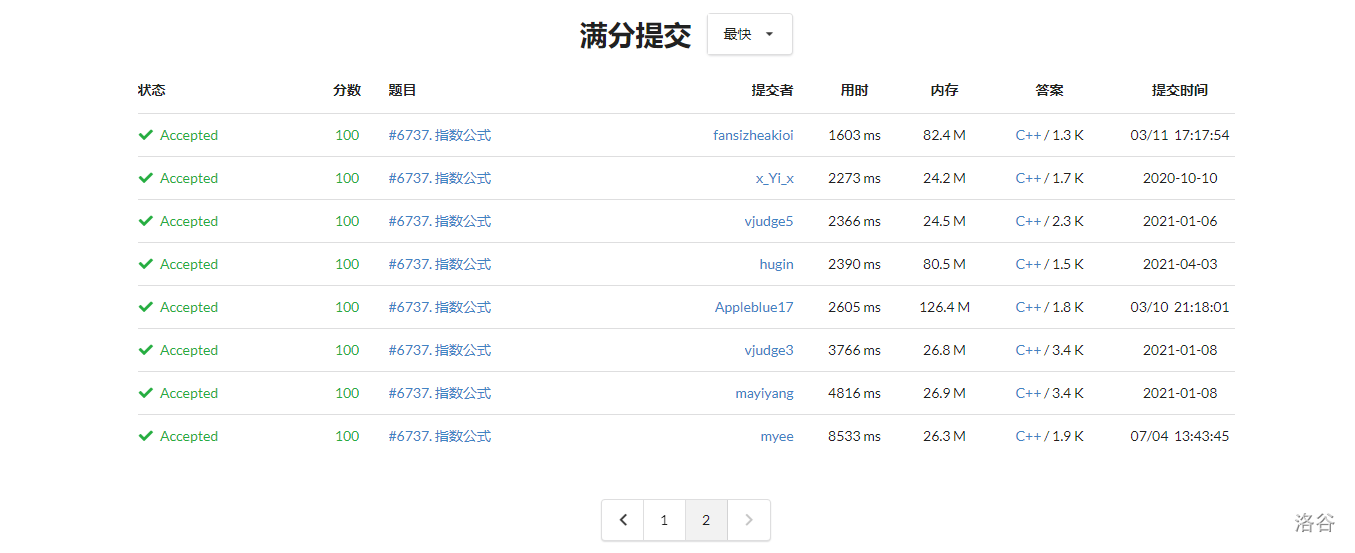# loj6737

2022-07-04 16:03:18  阅读：111  来源： 互联网

### 前言### Version 1

$$n\le 10^6$$，时限 $$1s$$。

$\binom ST=[T\subset S]$

$\exp F=\sum_n{F^n\over n!}$

### Version 2

$\exp\sum A=\prod\exp A$

### Version 3

$\texttt{Lemma}\ 1:\exp{z^n\over n!}=\sum_{k\ge0}{z^{nk}\over(nk)!}\prod_{t=1}^k\binom{nt-1}{n-1}$

$\texttt{Lemma}\ 2:2\nmid\binom{2n-1}{n-1}\Leftrightarrow\log_2n\in\mathbb N$

$\texttt{Lemma}\ 3:2\nmid[{z^m\over m!}]\exp{z^n\over n!}\Leftrightarrow(\log_2n\in\mathbb N\land n|m)\lor(\log_2n\notin\mathbb N\land(m=0\lor m=n))$

### Version 4

$\exp\sum_{k\in S}{z^k\over k!}=\prod_{k\in S}\exp{z^k\over k!}$

### Code

// Problem: #6737. 指数公式
// Contest: LibreOJ
// URL: https://loj.ac/p/6737
// Memory Limit: 256 MB
// Time Limit: 1000 ms

#include <algorithm>
#include <stdio.h>
#include <vector>
typedef long long llt;
typedef unsigned uint;typedef unsigned long long ullt;
typedef bool bol;typedef char chr;typedef void voi;
typedef double dbl;
template<typename T>bol _max(T&a,T b){return(a<b)?a=b,true:false;}
template<typename T>bol _min(T&a,T b){return(b<a)?a=b,true:false;}
template<typename T>T lowbit(T n){return n&-n;}
template<typename T>T gcd(T a,T b){return b?gcd(b,a%b):a;}
template<typename T>T lcm(T a,T b){return(a!=0||b!=0)?a/gcd(a,b)*b:(T)0;}
template<typename T>T exgcd(T a,T b,T&x,T&y){if(b!=0){T ans=exgcd(b,a%b,y,x);y-=a/b*x;return ans;}else return y=0,x=1,a;}
template<typename T>T power(T base,T index,T mod)
{
T ans=1%mod;
while(index)
{
if(index&1)ans=ans*base%mod;
base=base*base%mod,index>>=1;
}
return ans;
}
voi FMT(uint*A,uint n){for(uint i=1;i<n;i<<=1)for(uint j=0;j<i;j++)for(uint k=0;k<n;k+=i<<1)A[i|j|k]^=A[j|k];}
const uint Lim=1u<<21;
chr C[Lim|1];uint Now[Lim|1],A[Lim|1],B[Lim|1];
#define popcnt(v) __builtin_popcount(v)
int main()
{
scanf("%s",C+1);uint N=0;while(C[N+1])N++;
Now=A=A=1;
for(uint i=Lim>>1;i;i>>=1)
{
for(uint j=Lim/i-1;~j;j--)Now[j]=(j&1)?0:Now[j>>1];
if(C[i]=='1')FMT(Now,Lim/i);
}
for(uint i=0;i<Lim;i++)if(Now[i])Now[i]=1u<<popcnt(i);
for(uint w=4,q=2;w<=Lim;w<<=1,q++){
for(uint i=0;i<(w>>1);i++)A[i|(w>>1)]=A[i];
B=1;for(uint i=w>>1|1;i<w;i++)if(C[i]=='1')B[i]=1u<<popcnt(i);
FMT(B,w);
for(uint i=0;i<w;i++){
uint t=0;while(B[i])t^=A[i]*lowbit(B[i]),B[i]^=lowbit(B[i]);
A[i]=t;
}
}
FMT(Now,Lim);
for(uint i=0;i<Lim;i++)
{
uint t=0;while(Now[i])t^=A[i]*lowbit(Now[i]),Now[i]^=lowbit(Now[i]);
A[i]=t;
}
FMT(A,Lim);
for(uint i=1;i<=N;i++)putchar((A[i]>>popcnt(i)&1)+'0');
return 0;
}


2. 关于本站的所有留言、评论、转载及引用，纯属内容发起人的个人观点，与本站观点和立场无关；
3. 关于本站的所有言论和文字，纯属内容发起人的个人观点，与本站观点和立场无关；
4. 本站文章均是网友提供，不完全保证技术分享内容的完整性、准确性、时效性、风险性和版权归属；如您发现该文章侵犯了您的权益，可联系我们第一时间进行删除；
5. 本站为非盈利性的个人网站，所有内容不会用来进行牟利，也不会利用任何形式的广告来间接获益，纯粹是为了广大技术爱好者提供技术内容和技术思想的分享性交流网站。